# Cars plates

How many different licence plates can country have, given that they use 3 letters followed by 3 digits?

Result

n =  17576000

#### Solution:Leave us a comment of example and its solution (i.e. if it is still somewhat unclear...):Be the first to comment!#### To solve this example are needed these knowledge from mathematics:

See also our variations calculator. Would you like to compute count of combinations?

## Next similar examples:

1. Lock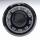Combination lock will open when the right choice of 5 numbers (from 1 to 12 inclusive) is selected. A. How many different lock combinations are possible? B. Is he combination lock named appropriately?
2. Bits, bytes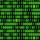Calculate how many different numbers can be encoded in 16-bit binary word?
3. MetalsIn the Hockey World Cup play eight teams, determine how many ways can they win gold, silver and bronze medals.
4. Football league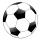In the 5th football league is 10 teams. How many ways can be filled first, second and third place?
5. Friends in cinema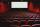5 friends went to the cinema. How many possible ways can sit in a row, if one of them wants to sit in the middle and the remaining's place does not matter?
6. Practice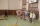How many ways can you place 20 pupils in a row when starting on practice?
7. MedalsIn how many ways can be divided gold, silver and bronze medal among 21 contestant?
8. Olympics metalsIn how many ways can be win six athletes medal positions in the Olympics? Metal color matters.
9. PIN - codesHow many five-digit PIN - code can we create using the even numbers?
10. Guests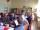How many ways can 5 guests sit down on 6 seats standing in a row?
11. Coin and die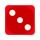Flip a coin and then roll a six-sided die. How many possible combinations are there?
12. 7 heroes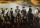9 heroes galloping on 9 horses behind. How many ways can sort them behind?
13. VariationsDetermine the number of items when the count of variations of fourth class without repeating is 42 times larger than the count of variations of third class without repetition.
14. A three-digit numbers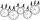Determine the total number of positive three-digit numbers that contain a digit 6.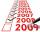Determine the number of integers from 1 to 106 with ending four digits 2006.We want to prove the sentence: If the natural number n is divisible by six, then n is divisible by three. From what assumption we started?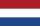From the colors - red, blue, green, black and white, create all possible tricolors.